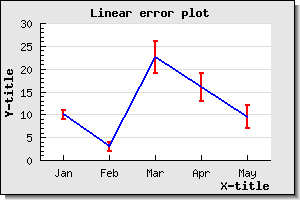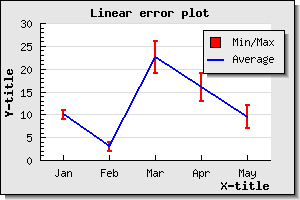## 15.3. Error plot graphs

Error plots are used to visually indicate uncertainty in data points. This is done by specifying both a min and and max value for each data point.

Before error plots can be used the module "`jpgraph_error.php`" must be included.

The following example illustrates a simple error bar. We will have 5 points, so we need 10 Y-values. We also would like the error bars to be red and 2 pixels wide. All this is accomplished by creating an instance of the `ErrorPlot` class in much the same way as, for example, a normal line plot.

Figure 15.58. A basic error plot `(example13.php)`There is one displeasing esthetic quality of this graph. The X-scale is just wide enough to just accompany the number of error bars and hence the first bar is drawn on the Y-axis and the and last bar just at the edge of the plot area.

To adjust this we can use the method `ErrorPlot::SetCenter()` which will adjust the x-scale so it does not use the full width of the X-axis.

Figure 15.59. Making use of SetCenter() with error plots `(example14.php)`### 15.3.1. Line error plots

A variant of the error plot graph is to use an `LineErrorPlot` instead. This is almost the same as the `ErrorPlot` but with the added feature that each data point also has an middle value which a line is drawn through. This can be thought of as a line plot combined with an error plot.

Since this also uses a line the module "`jpgraph_line.ph`p" must be included in addition to the error module.

To control the various properties of the line drawn the "line" property of the error line plot may be accessed. So, for example, to set the line to have weight of 2 pixels wide and with a blue color the following two lines are needed

 ```1 2 3 4 ``` ```line->SetWeight ( 2 ); \$elplot->line->SetColor ( 'blue' ); ?>```

An example of this is shown in Figure 15.60. We could now also add a legend to none, one or both of the line types(the plain line and/or the error bar). So for example if we wanted the legend "Min/Max" for the red error bars and a legend "Average" for the blue line the following lines should be added

 ```1 2 3 4 ``` ```SetLegend ( 'Min/Max' ); \$errplot->line->SetLegend ( 'Average' ); ?>```

The result is shown in Figure 15.61

 Figure 15.60. A basic Line error plot `(example15.php)`Figure 15.61. A line error plot with a legend `(example16.php)`